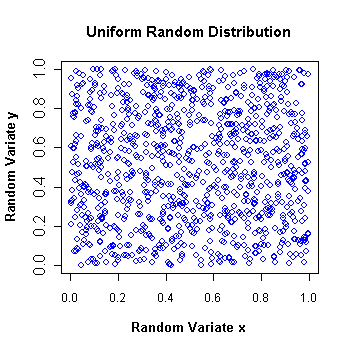I have forgotten
my Password

Or login with:

•http://facebook.com/
•https://www.google.com/accounts/o8/id
•https://me.yahoo.comCOST (GBP)1.980.000

# Mother Of All

Random number generator class using George Marsaglia's mother of all algorithm.
Controller: CodeCogsC++

## Class MotherOfAll

This class provides a long period random number generator using George Marsaglia's mother of all algorithm. It produces uniformly distributed pseudo-random 32-bit values with period of about George Marsaglia's original posting can be found at this link: ftp://ftp.taygeta.com/pub/c/mother.c

## Algorithm:

The arrays mother1 and mother2 store carry values in their first element and random 16-bit numbers in elements 1 to 8. These random numbers are moved to elements 2 to 9 and a new carry and number are generated and placed in elements 0 and 1. A 32-bit random number is obtained by combining the output of the two generators and returned in rnd. The arrays mother1 and mother2 are filled with random 16-bit values on the first call to Next().

To give you an idea of the running time for each of the functions, here are the results for generating 100,000,000 random numbers on a 750MHz microprocessor :
• genReal 16 seconds
• genInt 16 secondsnumbers in the (0, 1) interval and then plotting them one versus the other. It shows that the distribution is indeed uniform.

## References:

• George Marsaglia's original post of the mother of all generator algorithm, ftp://ftp.taygeta.com/pub/c/mother.c
• The Newran03 random number generator library of Robert Davies, http://www.robertnz.net/nr03doc.htm

### Example 1

The following example displays 20 random floating point numbers and 20 random large integers. It uses two different generators to achieve this. The first generator uses the system timer to initialize the seed, while the second is simply initialized with a particular value. Notice that it was necessary to divide the timer with the MOTHERDIV value in order to keep the seed in the (0, 1) interval. The output of the first generator will obviously vary with each execution of the program, while the output of the second will always be the same if the seed is never changed.
```#include <iostream>
#include <time.h>
#include <codecogs/statistics/random/motherofall.h>

using namespace std;

int main() {
Stats::Random::MotherOfAll A(time(0) / MOTHERDIV);
Stats::Random::MotherOfAll B(0.345);

for (int i = 0; i < 20; ++i)
cout << A.genReal() << endl;
cout << endl;

for (int i = 0; i < 20; ++i)
cout << B.genInt() << endl;
return 0;
}```

### Authors

Lucian Bentea (August 2005)
##### Source Code

Source code is available when you agree to a GP Licence or buy a Commercial Licence.

Not a member, then Register with CodeCogs. Already a Member, then Login.

## Members of MotherOfAll

#### MotherOfAll

 MotherOfAll( double s` = 0.46875` )[constructor]
 s Default value = 0.46875

#### SetSeed

 voidsetSeed( double s )
 s Seed must be in the (0, 1) interval (endpoints are excluded).